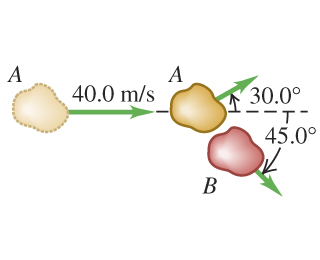# Problem: Two asteroids of equal mass in the asteroid belt between Mars and Jupiter collide with a glancing blow. Asteroid Aexttip{A}{A}, which was initially traveling at vA1 = 40.0 m/s with respect to an inertial frame in which asteroid  was at rest, is deflected 30.0° from its original direction, while asteroid B exttip{B}{B}travels at 45.0° ^circ to the original direction of Aexttip{A}{A}, as shown in the figure.(a) Find the speed of asteroid A after the collision.(b) Find the speed of asteroid B after the collision.(c) What fraction of the original kinetic energy of asteroid exttip{A}{A} dissipates during this collision?

🤓 Based on our data, we think this question is relevant for Professor Popov's class at UMICH.

###### Problem Details

Two asteroids of equal mass in the asteroid belt between Mars and Jupiter collide with a glancing blow. Asteroid A, which was initially traveling at vA1 = 40.0 m/s with respect to an inertial frame in which asteroid  was at rest, is deflected 30.0° from its original direction, while asteroid B travels at 45.0° to the original direction of A, as shown in the figure.
(a) Find the speed of asteroid A after the collision.
(b) Find the speed of asteroid B after the collision.
(c) What fraction of the original kinetic energy of asteroid dissipates during this collision?